##### The Japanese Bonsai specialist
Direct order Contact Help / Services Newsletter# Ophiopogon planiscapus 'nigrescens' 1 litre pot

Ophiopogon planiscapus nigrescens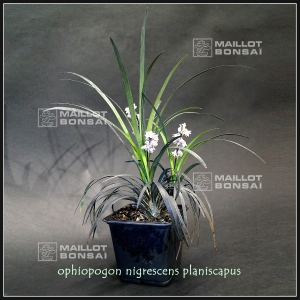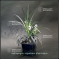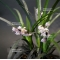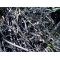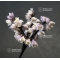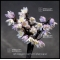ref. : 10509

7,00

Available quantity : 65Order

###### Description

Vivacious plant. Leaves 10/15-cm long and 0.4 cm wide. Strong tuft delivered in a 1 litre pot.

Ophiopogon planiscapus ‘Nigrescens’ is the “Black mondo grass”, an herbaceous perennial in the Asparagaceae family, and its grass-like blades are very black when it grows in full-sun. Small white-to-pink flowers bloom on leafless stems that rise above the foliage. Even if there are few, they are followed by glossy pea-sized purple berries. The name ophiopogon comes from Greek ophis meaning “serpent” and “pogon” meaning “beard”. Planiscarpus refers to the flattened scape or flowers stalk;

for example a tulip blooms a top a scape (from Latin scapus for “shaft”). Mondo grass is also commonly known as lilyturf, but the “mondo” name is an older genus name of unexplained origin, certainly not a japanese one. Ophiopogon’s name in Japan is , meaning “black dragon”.koku ryu

#ophiopogon 6 #name 4.4 #planiscapus 4.1 #meaning 3.7 #litre 3.5 #small 3.5 #grass 3.5 #nigrescens 3 #kusamono 2.8 #flowers 2.7

Formule
(( ROUND((CHAR_LENGTH(b.article_nom)-CHAR_LENGTH(REPLACE(b.article_nom, 'ophiopogon', '')))/LENGTH('ophiopogon')) + ROUND((CHAR_LENGTH(b.article_description)-CHAR_LENGTH(REPLACE(b.article_description, 'ophiopogon', '')))/LENGTH('ophiopogon')) ) * 6) + (( ROUND((CHAR_LENGTH(b.article_nom)-CHAR_LENGTH(REPLACE(b.article_nom, 'name', '')))/LENGTH('name')) + ROUND((CHAR_LENGTH(b.article_description)-CHAR_LENGTH(REPLACE(b.article_description, 'name', '')))/LENGTH('name')) ) * 4.4) + (( ROUND((CHAR_LENGTH(b.article_nom)-CHAR_LENGTH(REPLACE(b.article_nom, 'planiscapus', '')))/LENGTH('planiscapus')) + ROUND((CHAR_LENGTH(b.article_description)-CHAR_LENGTH(REPLACE(b.article_description, 'planiscapus', '')))/LENGTH('planiscapus')) ) * 4.1) + (( ROUND((CHAR_LENGTH(b.article_nom)-CHAR_LENGTH(REPLACE(b.article_nom, 'meaning', '')))/LENGTH('meaning')) + ROUND((CHAR_LENGTH(b.article_description)-CHAR_LENGTH(REPLACE(b.article_description, 'meaning', '')))/LENGTH('meaning')) ) * 3.7) + (( ROUND((CHAR_LENGTH(b.article_nom)-CHAR_LENGTH(REPLACE(b.article_nom, 'litre', '')))/LENGTH('litre')) + ROUND((CHAR_LENGTH(b.article_description)-CHAR_LENGTH(REPLACE(b.article_description, 'litre', '')))/LENGTH('litre')) ) * 3.5) + (( ROUND((CHAR_LENGTH(b.article_nom)-CHAR_LENGTH(REPLACE(b.article_nom, 'grass', '')))/LENGTH('grass')) + ROUND((CHAR_LENGTH(b.article_description)-CHAR_LENGTH(REPLACE(b.article_description, 'grass', '')))/LENGTH('grass')) ) * 3.5) + (( ROUND((CHAR_LENGTH(b.article_nom)-CHAR_LENGTH(REPLACE(b.article_nom, 'nigrescens', '')))/LENGTH('nigrescens')) + ROUND((CHAR_LENGTH(b.article_description)-CHAR_LENGTH(REPLACE(b.article_description, 'nigrescens', '')))/LENGTH('nigrescens')) ) * 3) + (( ROUND((CHAR_LENGTH(b.article_nom)-CHAR_LENGTH(REPLACE(b.article_nom, 'flowers', '')))/LENGTH('flowers')) + ROUND((CHAR_LENGTH(b.article_description)-CHAR_LENGTH(REPLACE(b.article_description, 'flowers', '')))/LENGTH('flowers')) ) * 2.7) + (( ROUND((CHAR_LENGTH(b.article_nom)-CHAR_LENGTH(REPLACE(b.article_nom, 'mondo', '')))/LENGTH('mondo')) + ROUND((CHAR_LENGTH(b.article_description)-CHAR_LENGTH(REPLACE(b.article_description, 'mondo', '')))/LENGTH('mondo')) ) * 2.5) + (( ROUND((CHAR_LENGTH(b.article_nom)-CHAR_LENGTH(REPLACE(b.article_nom, 'scape', '')))/LENGTH('scape')) + ROUND((CHAR_LENGTH(b.article_description)-CHAR_LENGTH(REPLACE(b.article_description, 'scape', '')))/LENGTH('scape')) ) * 2.5)

## Secure payment## Delivery

Our logistic partners :04 74 55 23 48
Pépinière MAILLOT-BONSAÏ
Le Bois Frazy
01990 RELEVANT - FRANCE
on appointment